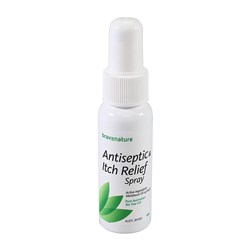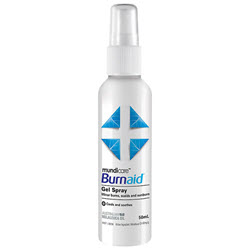# First Aid

Grid List
\$18.77
Increase value Decrease value
\$15.60
Increase value Decrease value
\$3.96
Increase value Decrease value
\$2.17
Increase value Decrease value
\$6.57
Increase value Decrease value
\$10.67
Increase value Decrease value
\$9.91
Increase value Decrease value
\$15.02
Increase value Decrease value
\$12.99
Increase value Decrease value\$8.95
Increase value Decrease value
\$17.07
Increase value Decrease value
\$31.57
Increase value Decrease value
\$8.48
Increase value Decrease value
\$4.73
Increase value Decrease value
\$1.39
Increase value Decrease value
Octenilin Wound Gel 20ml
\$31.83
Increase value Decrease value
\$39.85
Increase value Decrease value\$11.74
Increase value Decrease value
\$8.67
Increase value Decrease value
\$12.09
Increase value Decrease value
\$36.49
Increase value Decrease value
\$254.84
Increase value Decrease value
\$9.02
Increase value Decrease value
\$1.89
Increase value Decrease value
\$21.90
Increase value Decrease value
\$29.40
Increase value Decrease value
\$20.16
Increase value Decrease value
\$16.64
Increase value Decrease value
\$16.12
Increase value Decrease value
\$11.91
Increase value Decrease value
\$10.65
Increase value Decrease value
\$59.00
Increase value Decrease value
\$17.03
Increase value Decrease value
\$25.94
Increase value Decrease value
\$9.94
Increase value Decrease value
Hydrogen Peroxide 3% 100ml
\$8.27
Increase value Decrease value
\$12.36
Increase value Decrease value
\$7.83
Increase value Decrease value
\$16.81
Increase value Decrease value
\$249.00
Increase value Decrease value
\$16.92
Increase value Decrease value
\$14.19
Increase value Decrease value
\$25.38
Increase value Decrease value
\$33.62
Increase value Decrease value
\$0.91
Increase value Decrease value
\$9.20
Increase value Decrease value
\$15.17
Increase value Decrease value
\$126.10
Increase value Decrease value
Grid List
##### Products to compare:
Comparing Products